Courses

# NABARD Manager Quant Aptitude Mock Test 1

## 20 Questions MCQ Test NABARD Manager - Mock Tests & Previous Year Papers | NABARD Manager Quant Aptitude Mock Test 1

Description
This mock test of NABARD Manager Quant Aptitude Mock Test 1 for Banking Exams helps you for every Banking Exams entrance exam. This contains 20 Multiple Choice Questions for Banking Exams NABARD Manager Quant Aptitude Mock Test 1 (mcq) to study with solutions a complete question bank. The solved questions answers in this NABARD Manager Quant Aptitude Mock Test 1 quiz give you a good mix of easy questions and tough questions. Banking Exams students definitely take this NABARD Manager Quant Aptitude Mock Test 1 exercise for a better result in the exam. You can find other NABARD Manager Quant Aptitude Mock Test 1 extra questions, long questions & short questions for Banking Exams on EduRev as well by searching above.
QUESTION: 1

Solution:
QUESTION: 2

### The area of a square plot of twice the area of a rectangular plot. If the length of the rectangular plot is 36 metres and the area of the square plot is 1764 square metres. What is the breadth of the rectangular plot?

Solution:

Area of the square plot = 1764 square metre
According to the question,
Area of the rectangular plot
= 1764/ 2 = 882 square metre
Length of the rectangular plot = 36 metre
= 882 /36 metre = 24.5 metre

QUESTION: 3

### Two bicycles were sold for Rs 3990 each, gaining 5% on one and losing 5% on the other. The gain or loss per cent on the whole transaction is

Solution:
QUESTION: 4

In a school,average age of the students is 6 yrs and the average age of 12 teachers is 40 yrs. If the average age of the combined group of the 12 teachers and the students is 7 yrs,then the number of students is

Solution:

Let the no. of students be x
So sum of ages of students = 6x
Sum of ages of teachers = 12 x 40 = 480
Average age of combined group of 12 teachers and 'x' students is 7 years
i.e. 6x + 480 /x + 12 = 7
⇒ 6x + 480 = 7x + 84
⇒ x = 396

QUESTION: 5
An alloy contains copper and zinc in the ratio 5:3 and another alloy contains copper and tin in the ratio 8:5. If equal weights of both the alloys are melted together, then the weight of tin in the resulting alloy per kg will be
Solution:
QUESTION: 6
By giving a discount of 10% on the market price of Rs 1100 of a cycle, a dealer gains 10%.The cost price of the cycle is
Solution:
QUESTION: 7
Choose the correct alternative that will continue the same pattern and fill in the blank.
72, 76, 73, 77, 74, __, 75
Solution: This series alternates the addition of 4 with the subtraction of 3.
QUESTION: 8

A and B throw a dice. The probability that A's throw is not greater than B's is

Solution:

If B throws, 1, then A can throw only 1, if B throws 2, then A can throw 1 and 2 so on
∴ the required probability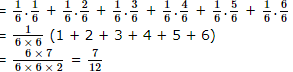QUESTION: 9
A sum of Rs 9000 is to be distributed among A, B and C in the ratio 4:5:6. What will be the difference between A's and C's shares?
Solution:
QUESTION: 10
What annual rate of interest was paid, if Rs 10000 earned Rs 1000 as interest in 2 yrs?
Solution:
QUESTION: 11

Rohit, Harsha and Sanjeev are three typists who, working simultaneously, can type 216 pages in four hrs. In one hr, Sanjeev can type as many pages more than Harsha as Harsha can type more than Rohit. During a period of five hrs, Sanjeev can type as many pages as Rohit can, during 7 hrs. How many pages does each of them type per hour?

Solution:

Suppose Rohit types x pages per hr
Harsha types y pages per hr
Sanjeev types z pages per hr
∴ z - y = y - x
⇒ y = x + z /2 .....(1)
⇒ 5z = 7x .....(2)
4(x + y + z) = 216
⇒ x + y + z = 54 .....(3)
Solving Eqs. (1), (2) and (3), we get
y = 18, x = 15, z = 21

QUESTION: 12

A swimming pool 9 m wide and 12 m long is 1 m deep on the shallow side and 4 m deep on the deeper side. Its volume is :

Solution:
QUESTION: 13

In the following questions two equations numbered I and II are given. You have to solve both the equations and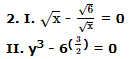Solution: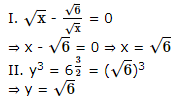QUESTION: 14

In the following questions two equations numbered I and II are given. You have to solve both the equations and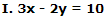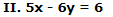Solution:

By equation I � 3 - equation II,
9x - 6y - 5x + 6y = 30 - 6
⇒ 4x = 24 ⇒ x = 6
From equation I,
3 � 6 - 2y = 10
⇒ 2y = 18 - 10 = 8
⇒ y = 4

QUESTION: 15

In which year was the increase in raw material maximum ?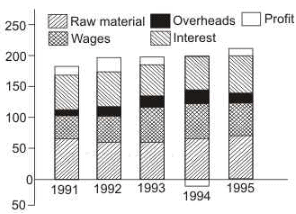Solution:
QUESTION: 16

Which component of the cost production has remained more or less constant over the period?Solution:

It is very clear that interest component remained more or less constant over the given period

QUESTION: 17

Percentage of students in Six different collegesTotal number of students = 3500
What is the number of girls in College D ?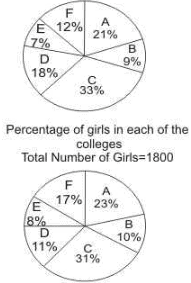Solution:
QUESTION: 18

Percentage of students in Six different colleges
Total number of students = 3500Which college has the lowest number of girls ?

Solution:

Total number of students = 3500
A = 735
B = 315
C = 1155
D = 630
E = 245
F = 420
Total number of girls = 1800
A = 414
B = 180
C = 558
D = 198
E = 144
F = 306
College E has 144 girls which is the lowest of all

QUESTION: 19

The total annul exports lay between 35 and 40 per cent to the total annual business of the IT industry, in the years :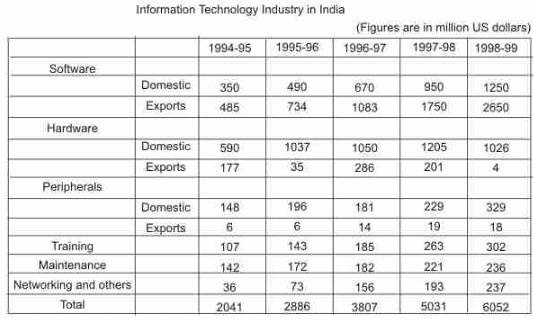Solution:
QUESTION: 20

The highest percentage growth in the total IT business, relative to the previous year was achieved in :Solution: